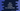# Java program to convert a string to an array of string### Introduction :

In this Java programming tutorial, we will learn how to convert a string to an array of strings. For example, if our input is Hello World, the output will be an array containing both of these words. We will show you two different and most popular ways to solve it.

### Using split() method :

split method takes one string as parameter. It creates an array of string by splitting the main string around matches of the given regular expression. In our case, we will pass blank space ” ” as a parameter. It will break the string in all blankspace parts and return us one array holding each word.

Source code :

``````import java.util.Arrays;

public class Main {
public static void main(String[] args) {
String myString = "Hello World ! This is me !!";

String[] arrayString = myString.split(" ");

System.out.println("Final array of string : " + Arrays.toString(arrayString));
}
}``````

#### Output :

``Final array of string : [Hello, World, !, This, is, me, !!]``

As you can see that the final array holds each word of the given string. To print a word in the array, we can use its specific index like arrayString to print the first word “Hello”.

### Using regex.Pattern class :

We have one more different method to solve this problem: by using regex.Pattern class. The process is similar to the above. First, create one Pattern object with a regular expression blank space (” ”). Then, split the string using the created pattern. Source code :

``````import java.util.Arrays;
import java.util.regex.Pattern;

public class Main {
public static void main(String[] args) {
String myString = "Hello World ! This is me !!";

Pattern pattern = Pattern.compile(" ");
String[] arrayString = pattern.split(myString);

System.out.println("Final array of string : " + Arrays.toString(arrayString));
}
}``````

#### Output :

``Final array of string : [Hello, World, !, This, is, me, !!]``